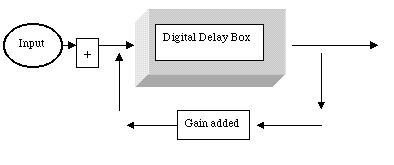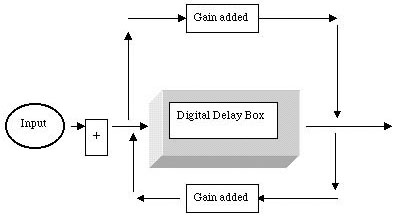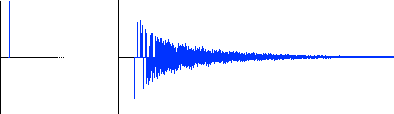<-- Back to Previous Page TOC Next Section --># Section 5.2: Reverb

One of the most important and widely used techniques in computer music is reverberation and the addition of various types and speeds of delays to dry (unreverberated) sounds. Reverberation and delays can be used to simulate room and other environmental acoustics or even to create new sounds of their own that are not necessarily related to existing physical spaces.

There are a number of commonly used techniques for simulating and modeling different reverberations and physical environments. One interesting technique is to actually record the ambience of a room and then superimpose that onto a sound recorded elsewhere. This technique is called convolution.

## A Mathematical Excursion: Convolution in the Time Domain

Convolution can be accomplished in two equivalent ways: either in the time domain or in the frequency domain. We’ll talk about the time-domain version first. Convolution is like a "running average"—that is, we take the original function representing the music (we’ll call that one m(n), where m(n) is the amplitude of the music function at time n) and then we make a new, smoothed function by making the amplitude a at time n of our new function equal to:

a = (1/3)[m(n – 2) + m(n – 1) + m(n)]

Let’s call this new function r(n)—for running average—and let’s look at it a little more closely.

Just to make things work out, we’ll start off by making r(0) = 0 and r(1) = 0. Then:

r(2) = 1/3[m(0) + m(1) + m(2)]

r(3) = 1/3[m(1) + m(2) + m(3)]

We continue on, running along and taking the average of every three values of m— hence the name "running average." Another way to look at this is as taking the music m(n) and "convolving it against the filter a(n)" where a(n) is another function, defined by:

a(0) = 1/3, a(1) = 1/3, a(2) = 1/3

All the rest of the a(n) are 0.

Now, what is this mysterious "convolving against" thing? Well, first off we write it like:

m*a(n)

then:

m*a(n) = m(n)a(0) + m(n – 1)a(1) + m(n – 2)a(2) +....+ m(0)a(n)

next:

m*a(n) = m(n)(1/3) + m(n – 1)(1/3) + m(n – 2)(1/3)

Now, there is nothing special about the filter a(n)—in fact it could be any kind of function. If it were a function that reflects the acoustics of your room, then what we are doing—look back at the formula—is shaping the input function m(n) according to the filter function a(n). A little more terminology: the number of nonzero values that a(n) takes on is called the number of taps for a(n). So, our running average is a three-tap filter.

## Reverb in the Time Domain

Convolution is sure fun, and we’ll see more about it in the following section, but a far easier way to create reverb in the time domain is to simply delay the signal some number of times (by some very small time value) and feed it back onto itself, simulating the way a sound bounces around a room. There are a large variety of commercially available and inexpensive devices that allow for many different reverb effects. One interesting possibility is to change reverb over the course of a sound, in effect making a room grow and shrink over time (see Soundfile 5.7).Soundfile 5.7
Reverberant characteristics

Changing the reverberant characteristics of a sound over time.

Here is a nail being hammered. The room size goes from very small to very large over the course of about 15 seconds. This can’t happen in the real, physical world, and it’s a good example of the way the computer musician can create imaginary soundscapes.

Before there were digital systems, engineers created various ways to get reverb-type effects. Any sort of situation that could cause delay was used. Engineers used reverberant chambers—sending sound via a loudspeaker into a tiled room and rerecording the reverb-ed sound. When one of us was a kid, we ran a microphone into our parents' shower to record a trumpet solo!

Reverb units made with springs and metal plates make interesting effects, and many digital signal processing units have algorithms that can be dialed up to create similar effects. Of course we like to think that with a combination of delayed copies of signals we can simulate any type of enclosure, whether it be the most desired recording studio room or a parking garage.

As you’ll see in Figure 5.5, there are some essential elements for creating a reverberant sound. Much of the time a good reverb "patch," or set of digital algorithms, will make use of a number of copies of the original signal—just as there are many versions of a sound bouncing around a room. There might be one copy of the signal dedicated to making the first reflection (which is the very first reflected sound we hear when a sound is introduced into a reverberant space). Others might be algorithms dedicated to making the early reflections—the first sounds we hear after the initial reflection. The rest of the software for a good digital reverb is likely designed to blend the reverberant sound. Filters are usually used to make the reverb tails sound as if they are far away. Any filter that attenuates the higher frequencies (like 5 kHz or up) makes a signal sound farther away from us, since high frequencies have very little energy and don’t travel very far.

When we are working to simulate rooms with digital systems, we have to take a number of things into consideration:

• How large is the room? How long will it take for the first reflection to get back to our ears?
• What are the surfaces like in the room? Not just the walls, ceiling, and floor, but also any objects in the room that can reflect sound waves; for example, are there any huge ice sculptures in the middle of the room?
• Are there surfaces in the room that can absorb sound? These are called absorptive surfaces. For example: movie theaters usually have curtains hanging on the walls to suck up sound waves. Maybe there are people in the room: most bodies are soft, and soft surfaces remove energy from reflected sounds.Figure 5.5  Time-domain reverb is conceptually similar to the idea of physical modeling—we take a signal (say a hand clap) and feed it into a model of a real-world environment (like a large room). The signal is modified according to our model room and then output as a new signal.

In this illustration you see the graphic representation of a direct sound being introduced into a reverberant space. The RT 60 refers to reverb time, or how long it takes the sound in the space to reduce by –60 dB.

Photo courtesy of Garry Clennell <gcat@clara.co.uk>.Figure 5.6  In this illustration you can see how sound from a sound source (like a loudspeaker) can bounce around a reverberant space to create what we call reverb.

A typical reverb algorithm can have lots of control variables such as room size (gymnasium, small club, closet, bathroom), brightness (hard walls, soft walls or curtains, jello), and feedback or absorption coefficient (are there people, sand, rugs, llamas in the room—how quickly does the sound die out?).

Photo courtesy of Garry Clennell <gcat@clara.co.uk>.Figure 5.7  Here’s a basic diagram showing how a signal is delayed (in the delay box), then fed back and added to the original signal with some attenuated (that is, not as loud) version of the original signal added. This would create an effect known as comb filtering (a short delay with feedback that emphasizes specific harmonics) as well as a delay.

What does a delay do? It takes a function (a signal), shifts it "backward" in time, then combines it with another "copy" of the same signal. This is, once again, a kind of averaging.Figure 5.8  When we both feed-back and feed-forward the same signal at the same time, phase inverted, we get what is called an all-pass filter. (Phase inverting a signal means changing its phase by 180°.) With this type of filter/delay, we don’t get the comb filtering effect because of the phase inversion of the feed-forward signal, which fills in the missing frequencies created by the comb filter of the feedbacked signal.

This type of unit is used to create blended reverb. A set of delay algorithms would probably have some combination of a number of comb-type delays as in this figure, as well as some all-pass filters to create a blend.

As we mentioned, convolution is a great way to get reverberant effects. But we mainly talked about the mathematics of it and why convolving a signal is basically putting it through a type of filter.

But now we’re going to talk about how to actually convolve a sound. The process is fairly straightforward:

1. Locate a space whose reverberant characteristics we like.
2. Set up a microphone and some digital media for recording.
3. Make a short, loud sound in this room, like hitting two drumsticks together (popping a balloon and firing a starter’s pistol are other standard ways of doing this—sometimes it’s called "shooting the room").
4. Record this sound, called the impulse.
5. Analyze the impulse in the room; this gives us the impulse response of the room, which is the characteristics of the room’s response to sound.
6. Use the technique of convolution to combine this impulse response with other sound sources, more or less bringing the room to the sound.

Convolution can be powerful, and it’s a fairly complicated software process. It takes each sample in the impulse response file (the one we recorded in the room, which should be short) and multiplies that sample by each sample in the sound file that we want to "put in" that room. So, each sample input sound file, like a vocal sound file to which we want to add reverb, is multiplied by each sample in the impulse response file.

That’s a lot of multiplies! Let’s pretend, for the moment, that we have a 3-second impulse file and a 1-minute sound file to which we want to add the reverberant characteristics of some space. At 44.1 kHz, that’s:

`3 * 44,100 * 60 * 44,100`

`= 180 * 680,683,500,000 `

`= 122,523,403,030,000 multiplies`

If you’ve been paying close attention, you might raise an interesting question here: isn’t this the time domain? We’re just multiplying signals together (well, actually, we’re multiplying each point in each function by every other point in the other function—called a cross multiply). But this multiply in the time domain actually produces what we refer to as the convolution in the frequency domain.

Now, we’re not math geniuses, but even we know that this is a whole mess of multiplies! That’s why convolution, which is very computationally expensive, had not been a popular technique until your average computer got fast enough to do it. It was also a completely unknown sound manipulation idea until digital recording technology made it feasible.

But nowadays we can do it, and the reason we can do it is our old friend, the FFT! You see, it turns out that for filters with lots of taps (remember that this means one with lots of nonzero values), it is easier to compute the convolution in the spectral domain.

Suppose we want to convolve our music function m(n) against our filter function a(n). We can tell you immediately that the convolution of these functions (which, remember, is another sound, a function that we call c(n)) has a spectrum equal to the pointwise product of the spectrum of the music function and the filter function. The pointwise product is the frequency content at any point in the convolution and is calculated by multiplying the spectrums of the music function and the filter function at that particular point.

Another way of saying this is to say that the Fourier coefficients of the convolution can be computed by simply multiplying together each of the Fourier coefficients of m(n) and a(n). The zero coefficient (the DC term) is the product of the DC terms of a(n) and m(n); the first coefficient is the product of the first Fourier coefficient of a(n) and m(n); and so on.

So, here’s a sneaky algorithm for making the convolution:

Step 1: Compute the Fourier coefficients of both m(n) and a(n).

Step 2: Compute the pointwise products of the Fourier coefficients of a(n) and m(n).

Step 3: Compute the inverse Fourier transform (IFFT) of the result of Step 1.

And we’re done! This was one of the great properties of the FFT: it made convolution just about as easy as multiplication!

So, once again, if we have a big library of great impulse responses— the best-sounding cathedrals, the best recording studios, concert halls, the Grand Canyon, Grand Central Station, your shower—we can simulate any space for any sound. And indeed this is how many digital effects processors and reverb plugins for computer programs work. This is all very cool when you’re working hard to get that "just right" sound.Figure 5.9  The impulse on the left, and the room’s response on the right.

Thanks to Nigel Redmon of earlevel.com for this graphic.

 <-- Back to Previous Page Next Section -->